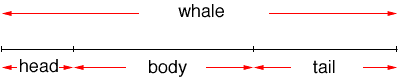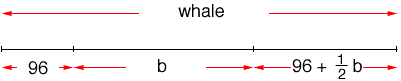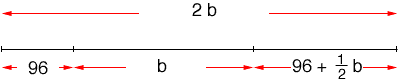Quandaries and Queries I found a problem in the Quandaries and Queries about " A whale's head is 72 inches long... its tail is as long as its head plus half the length of its body, and its body is half the entire length. Who long is the whale?" You have given a formula for this: 72+2x+72+x=2(2x). I'm sorry to say that I still didn't get it. . Could you please explain this to me in the simplest terms possible, step by step, and could you please substitute 9 instead of 72. Thanks Judy Hi Judy, Since it wasn't clear to you the way I did it I am going to doo something slightly different. Also 9 inches is really small for the length of a whale's head so let me do the problem if the length of the head is 96 inches. When I read the problem I see that the whale is described in terms of its head, its body and its tail. So what I see isI know that the head is 96 inches long and the problem also says its tail is as long as its head plus half the length of its body so if I knew the length of the body I would be able to find the length of the tail. I can say this more explicitly if I let the length of the body be b then this fact says tail length = 96 + 1/2 b I want to put this information on the diagramThe other fact given is body is half the entire length so b is half the entire length of the whale and thus the entire length is 2b. Thus, on the diagramHence 2b = 96 + b + 96 + 1/2 b Solve for b then the length of the whale is 2b. Penny Go to Math Central